New Functions - GENERATESERIES, SELECTEDVALUE - Excelerator BI

# New Functions – GENERATESERIES, SELECTEDVALUE

Level: Beginners/Intermediate

In the August 2017 update of Power BI Desktop Microsoft released the new What If feature (first demonstrated at MDIS in July).  This new What If capability uses two new DAX functions to complete a task that you have always been able to do manually.  You still can of course still do it manually, but now it is easier than ever as the new functions make the task of creating a disconnected table/slicer very easy indeed.  I am not actually sure if these 2 new functions were created in order to make the What If process easier or if that was a co-incidence.  Regardless, let me explain the new underlying DAX functions and how they work so you can use these without the What If button if you choose.

Both of the new functions are currently only available in Power BI (to my knowledge).  I know for sure they are not currently available in Excel.

## The Old Way

I have been doing what the new What If tool does manually for years (ie allow a slicer to pass possible values to a measure as input).  The old way (still the current way if you are using Power Pivot for Excel) is as follows:

• Create a list of values you want to pass as a what if input to a DAX measure.
• Load that list of values to a table in the data model.  Don’t connect the table to any other table
• Create a “harvester measure” to “harvest” which value has been selected in the slicer by the user.
• Use the value returned by the harvester measure inside another measure so you can test out various scenarios.

The new What If tool provides an automated (and easier) way to complete this process. Today I am going to cover the 2 new underlying DAX functions that are automatically used by the What If button to streamline this process.

## GENERATESERIES()

This new function is used to generate the table of possible values – it has the following syntax:

GENERATESERIES(StartValue, EndValue, [IncrementValue])

The function returns a table, therefore if you are going to use this function manually you either need to use the New Table button (which can return a table), or alternatively (potentially) you could use it as a table input into another DAX function.  I have an idea in my head where you could write a function that compounds interest over a number of years by cycling through such a virtual table, without ever materialising the table itself. Anyway, I digress – let’s look at the new function.

First let me create a sample table to show how it works.  To do this I clicked on New Table in Power BI Desktop and then added the following DAX formula.

myTable = GENERATESERIES(1,10,0.1)

This created the following table (containing 91 rows)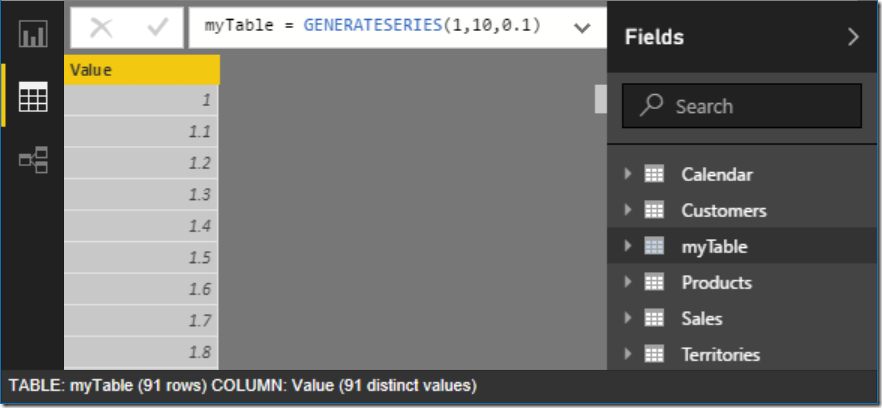The column name VALUE is automatically applied to the column, but you can rename it if you don’t like this default name.

### UNION Solves a Problem

The biggest “gap” I see with this approach vs “the old way” is that each value is equally spaced by the same ‘increment’ amount.  But what if you want to have a table from 1..10 with a step of 1, then 20..100 with a step of 10?  Well actually this is pretty easy.  To do this, first create DAX for a second table as follows:

GENERATESERIES(20,100,10)

Then combine the DAX for both tables into a single New Table using a UNION function as follows:

myNewTable = UNION(GENERATESERIES(1,10,1),GENERATESERIES(20,100,10))

This all works fine and as expected.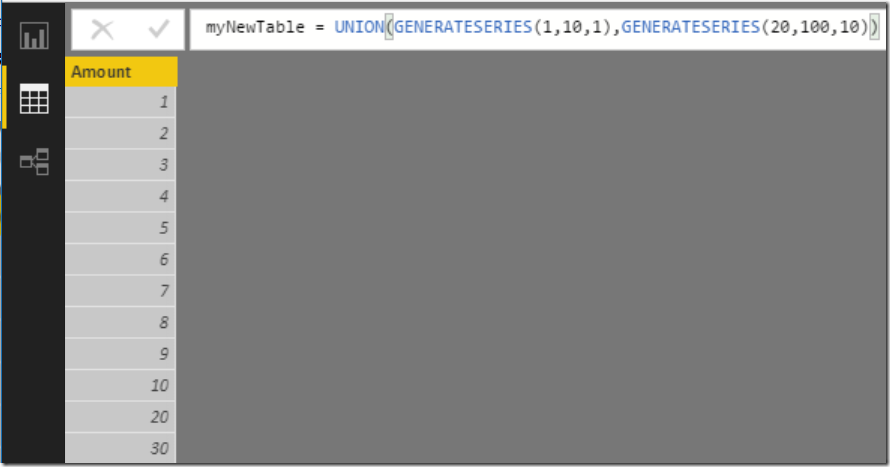You can join/append as many tables as you like using UNION to create non sequential number range.

### UNION vs UNION ALL

It is worth pointing out here that UNION in DAX is the same as UNION ALL in SQL.  Consider the following DAX formula

myNewTable = UNION(GENERATESERIES(1,10,1),GENERATESERIES(10,100,10))

When I combine these using UNION in DAX, I get the table below – see in the image below that the table has the value 10 twice.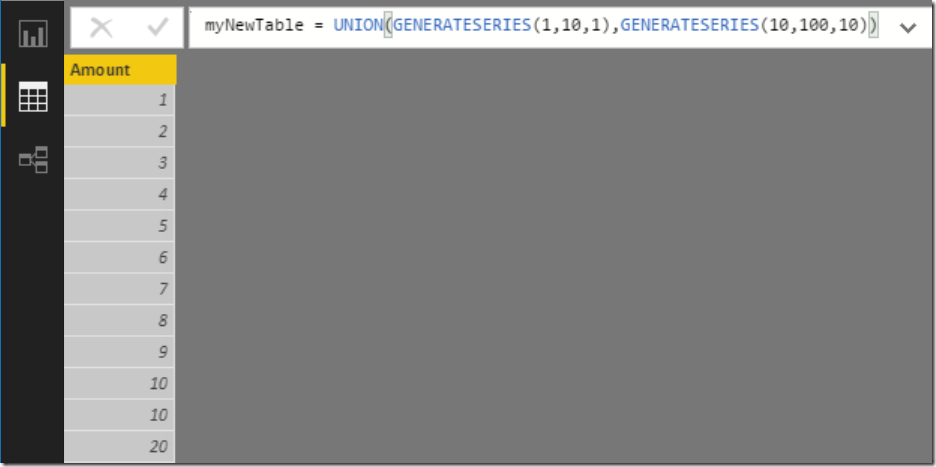This is easy to fix by wrapping the entire UNION table function inside a DISTINCT function as below.

myNewTable = DISTINCT(UNION(GENERATESERIES(1,10,1),GENERATESERIES(10,100,10)))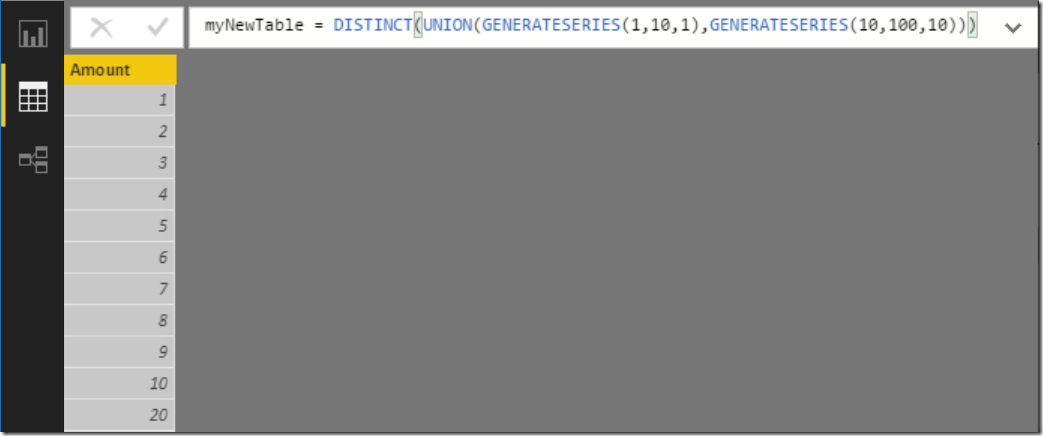If you don’t remove the duplicates, you may be undesired behaviour.## SELECTEDVALUE()

This new function lets you “check” which value is selected by a user in a table (like the one above) – it has other uses too.  Rob Collie coined the term “Harvester Measure” for these little suckers.  But before I cover this new function, first let me talk about the VALUES() function.  VALUES() returns a single column table of all the values in the current filter context.  But it also has a very special property.  If there is 1 and only 1 value selected in the current filter context, then you can display that value in a card or any other visual.  But you must first protect the visual against the case where there is more than 1 value in the VALUES() table.  To protect against this, you must use the following construct

=IF(HASONEVALUE(table[Column]),VALUES(Table[Column]))

The IF/HASONVALUE is simply protection against this undesired scenario (in this case) where the formula returns more than one value (hence it automatically turns back into a table – that is bad).

The new function SELECTEDVALUE() is simply a replacement for this cumbersome construct above (syntax sugar I assume).  The syntax of the new function is as follows

SELECTEDVALUE(table[Column],[AlternateResult]).  If the optional alternate result is omitted, the function will return BLANK() in the instance where there is something other than 1 selected value in the current filter context.  See the example below.

SelectedValue = SELECTEDVALUE(myNewTable[Value]).  Note below there is nothing selected in the slicer and hence the card returns (Blank).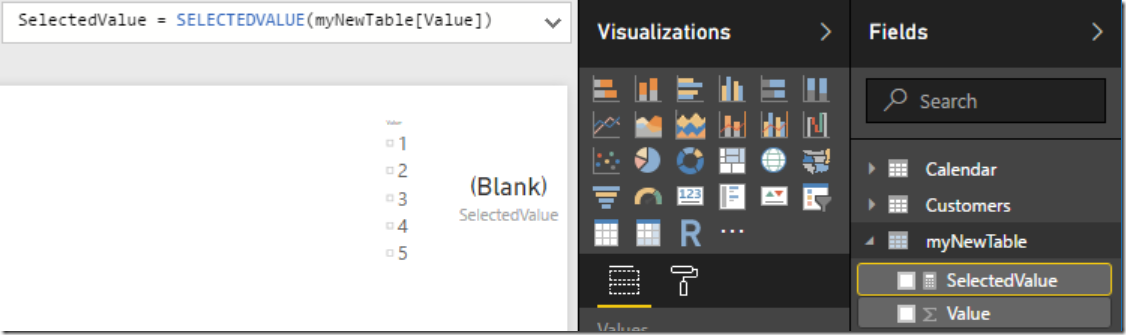In the instance where there is a single value selected, that value is returned to the visual (a card in this case as shown below).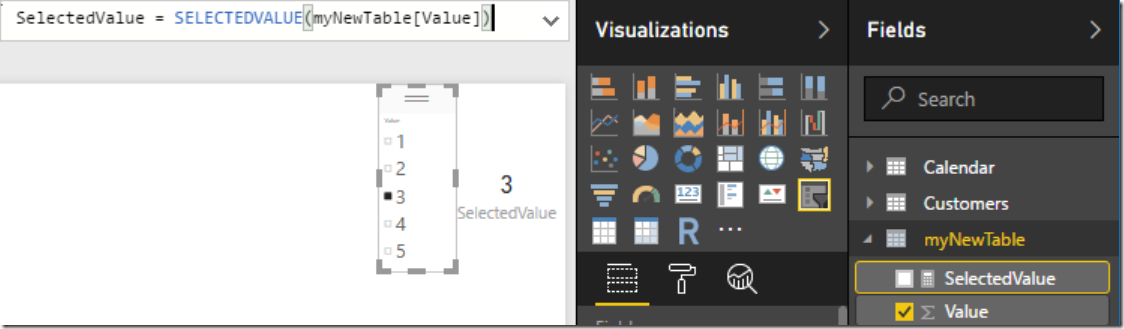## The What If Button

The new What If Button simply produces a new table using the GENERATESERIES() function and also produces a new measure to display the single selected value using the SELECTEDVALUE() function.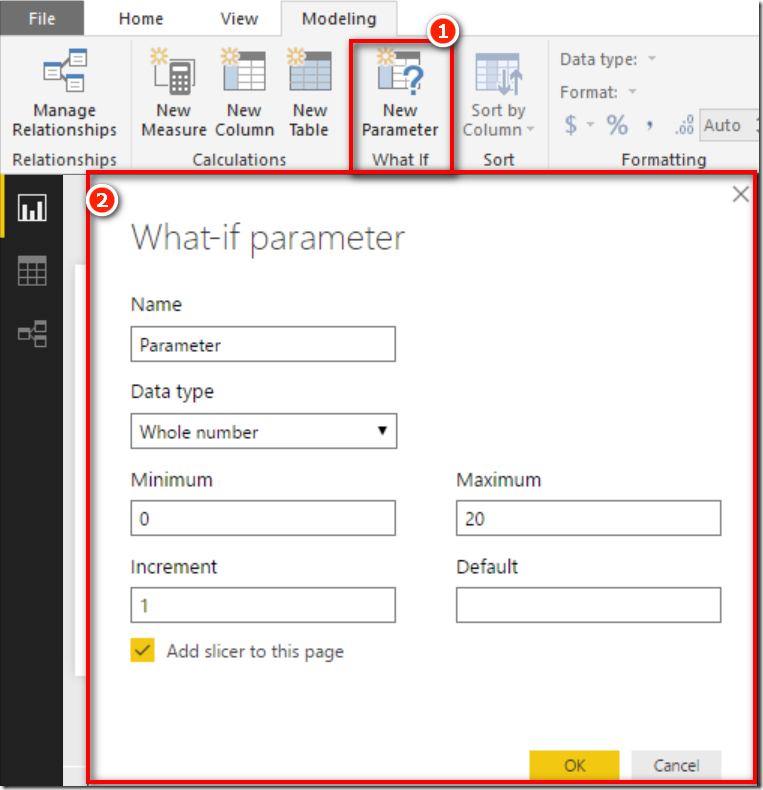This is yet another step towards making Power BI accessible to people that are not familiar with DAX.  A word of caution however (as always).  The more of these “make it easy” features that are delivered, the more you will get into the detail before realising you don’t really understand the concepts of filter context, row context and context transition.  To learn this properly, there is no substitute for some formal learning in either a live class, an online class or a good book.

## DAX Quick Reference Guide

### 11 thoughts on “New Functions – GENERATESERIES, SELECTEDVALUE”

1.Thnx Matt, this is great to customise my reports using parameters.

Quick question:
How do I save these selected-values from multiple lists into a set of variables/parameters so that I can use them in my entire reporting system.
Eg: If I use #3 as my value above, it would be used in all DAX formuals. Hence if I change selected-value to #4, then DAX formula gets picked the new value?

1.I’m not sure I fully follow. If you select a different value in the slicer, then the underlying table is filtered. It will stay in that state until you change the slicer. So any measure that points to that table will change with the slicer change. Does that clarify it?

2.Matt,
for me the GENERATESERIES function returns wrong results when used with decimals in a calculated table.
e.g. CalculatedTable = GENERATESERIES(0.1, 1, 0.1) returns a table corresponding to {0,0,0,0,1,1,1,1,1,1}
Maybe, it’s my German version having problems with the decimal seperator.
Does it work for you?

1.That looks like a rounding problem to me. What is the number format of the column? It looks like integer – try one decimal

1.You are right, I started with integers and then switched to decimals. Thanks!

3.Hi Matt,

Nice post. You are shortly hinting towards creating a measure for interest. I am very interested in that; I want to include a price-index parameter in my report. The index needs to increase over time (e.g 2% index => 1,02 in 2018; 1,02×1,02 in 2019, 1,02×1,02×1,02 in 2020 etc.) so that future prices are increased with 2% every year.

Can you give a direction how to do this?

1.I hadn’t really thought this through fully hence me half baked comment. Maybe it is not a virtual table after all (now I think about it). If you materialised the table using GENERATE series, you could add that table as an input to a matrix (on row).
GENERATESERIES(1,5,1).
Then the future value would be = (1+[interest rate])^SELECTEDVALUE(table[value])

4.Hi Matt! Wonderful post. I am reading The Definitive Guide to DAX and today morning I was completing Row / Filter contexts and relationships, VALUES and Creating parameter table pages. I could find similarity between these pages and this article. Thank you a lot for spending your time helping the rest of the world with DAX.

5.Matt, Good post. I think it’s great for Power BI adaptation that these functions are created as they will cover 90% of standard requirements. I question the value of these new functions as the creation of a disconnected slicer and creation of harvester measure may have been manual but, it wasn’t tedious and certainly allowed for a more nuanced granularity.

6.Thanks for explaining the two new functions, Matt.

7.Nice post, Matt! I’m glad to see that you pointed out using Union with Generateseries, along with some things to look for.

Scroll to Top